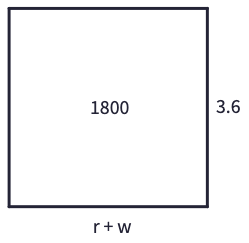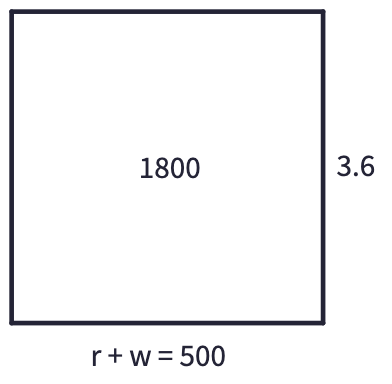This is a tough question. There are so many things I'd like to have done differently and many things I would not change. If I am thinking about my teenage self and math I guess I would advise myself to not be so afraid. To be more confident with my words. To demand help when I needed it rather than ask, be denied, and quit math because of it. Also, I would tell myself just how fun math is and that math is not just about answers. Math is about problem-solving. It is not about being fast but rather about being steady and having stamina. I would tell myself how much enjoyment there is in working through those really hard problems even when they take hours, days, or weeks to figure out. I would tell myself that one day I will get a kick out of solving math problems for fun. Not because I have to, but because I want to. That there is satisfaction in finding easier more unique ways to problem solve than what is traditionally taught. I would show myself that solving math problems are no problem.

Case in point: An airplane travels 1800 miles from LA to Chicago in 3.6 hours, going with the wind. The return trip takes 4 hours and is against the wind. Find the rate of the airplane and the rate of the wind.

Solving this problem is as simple as drawing rectangles and labelling the sides to get a simple system of equations to work with.r = rate of the airplane in miles/hour
t = time in hours
d = distance in miles
w = rate of the wind in miles/hour
r + w = airplane with the wind
r - w = airplane against the wind(r + w)(3.6) = 1800
(r + w)(3.6) = (500)(3.6)
r + w = 500(r − w)(4) = 1800
(r − w)(4) = (450)(4)
r − w = 450r + w + r - w = 500 + 450
r + r + w - w = 950
2r + 0 = 950

2r = 950
2r = (2)(475)
r = 475r + w = 500
475 + w = 500
475 + w = 475 + 25
w = 25r − w = 450
475 − w = 450
475 − w = 475 − 25
w = 25(r + w)(3.6) = 1800
(475 + 25)(3.6) = 1800
(500)(3.6) = 1800(r − w)(4) = 1800
(475 − 25)(4) = 1800
(450)(4) = 1800

rate of airplane =
(475 miles)/hour

rate of wind =
(25 miles)/hour

Math is the study of numbers.

All we do with numbers is count them.

When we need a "Hero" call on "Zero".

If it's no fun get back to "One".

= means same as.

Imagination, not memorization.

Categories: Tags: